Courses

# Test: Alternating Current- 2

## 25 Questions MCQ Test Physics For JEE | Test: Alternating Current- 2

Description
This mock test of Test: Alternating Current- 2 for Class 12 helps you for every Class 12 entrance exam. This contains 25 Multiple Choice Questions for Class 12 Test: Alternating Current- 2 (mcq) to study with solutions a complete question bank. The solved questions answers in this Test: Alternating Current- 2 quiz give you a good mix of easy questions and tough questions. Class 12 students definitely take this Test: Alternating Current- 2 exercise for a better result in the exam. You can find other Test: Alternating Current- 2 extra questions, long questions & short questions for Class 12 on EduRev as well by searching above.
QUESTION: 1

### In an a.c. circuit voltage V and current i are given by V = 100 sin 100 t volts, i = 100 sin (100t + p/3) mA. The power dissipated in the circuit is :

Solution:

Vrms = 100/√2 V
Irms = 100/√2 mA
= 10-1/√2 A
π/3 = 30º
Average power = Vrms×Irms× cos
= (100/√2)×(10-1√2)× cos 30º
= 10/2×(1/2)
= 10/4
= 2.5W

QUESTION: 2

### The potential difference V and current i flowing through an a.c. circuit are given by V = 5 cos wt volt, i = 2 sin wt amp. the power dissipated in the circuit.

Solution:

The voltage can be written as,
V=5[sinωt+(π/2​)]
The angle between the voltage and the current is 90∘.
The power dissipated in the circuit is given as,
P=Vrms​Irms​cosϕ
=Vrms​Irms​cos90o
=0
Thus, the power dissipated in the circuit is 0.

QUESTION: 3

### An a.c. circuit consists of an inductor of inductances 0.5 H and a capacitor of capacitance 8 mF in series. The current in the circuit is maximum when the angular frequency of an a.c. source is

Solution:

Given,
Inductance and capacitance are joined in series, and inductance L=0.5H,
And capacitance C=8μF=8×10−6F.
We know that,
ω=1/√(LC)
ω=1/√(8×10−6×0.5)=1/(2×10−3)=0.5×103HZ=500HZ

QUESTION: 4

The rms value of an AC of 50 Hz is 10 amp. The time taken by an alternating current in reaching from zero to maximum value and the peak value will be ;

Solution:

Time taken by alternating current to reach maximum from zero is 4T​.
where T is the time period of the AC function.
T=1/f​, Frequency (f)=50 Hz.
So time taken to reach maximum=T/4​=1/4f​=1/(4×50)​=5×10−3 sec
IRMS​=​I0/√2​, I0​=Peak value of current.
Peak value of current I0​=√2​×IRMS​=√2​×10=14.14 A

QUESTION: 5

A voltage of peak value 283 V varying frequency is applied to a series L-C-R combination in which R = 3W; L = 25 mH and C = 400 mF. Then, the frequency (in Hz) of the source at which maximum power is dissipated in the above, is

Solution:

Here, V0​=283V,R=3Ω,L=25×10−3H
C=400μF=4×10−4F
Maximum power is dissipated at resonance, for which

ν=1/[2π√(LC)]​​= (1×7​)/[2×22(25×10−3×4×10−4)]
=(7×103​)/(44√10​)
=50.3Hz

QUESTION: 6

The power factor of the circuit is 1/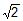. The capacitance of the circuit is equal to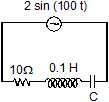Solution:

Here, it is given that,
power factor in the circuit, = 1/√2
Resistance, R = 10Ω
inductance, L = 0.1 H
Supply voltage, V = 2sin(100t)
peak voltage, V₀ = 2
capacitance = ?
=> power factor = cos∅ = R/Z
1/√2 = 10/Z
=> By Taking the square on both the sides,
1/2 = 100/Z2
Z2 = 200
=> Now, we can use below formula to calculate the capacitance of the circuit.
R2 + (Xc - XL)2 = Z2
10² + (1 / cω - Lω)² = 200
(1/cω - Lω)2 = 100
1/cω - Lω = 10
1/cω = Lω + 10
1/cω = 0.1 * 100 + 10
1/cω = 20
c = 1/(20xω)
c = 1/(20x100)
c = 1/2000 F
c = 106 / 2000 (μ)F    [∵ 1 F = 106 (μ)F ]
= 500 (μ) F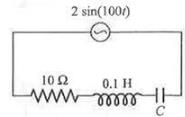QUESTION: 7

An ac-circuit having supply voltage E consists of a resistor of resistance 3W and an inductor of reactance 4W as shown in the figure. The voltage across the inductor at t = p/w is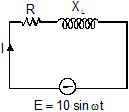Solution:

Here,
XL = 4 Ω
R = 3 Ω
Z = √(XL2 + R2)=√(42 + 32)
= 5 Ω
E0 = 10 V
In the LR circuit current in the ckt is given by
I = (E0/Z)sin(ωt - Φ)
Φ = tan-1(XL/R)
=>  Φ = tan-1(4/3)
I = (E0/Z)sin(ωt - Φ)
= (10/5) sin(ωt - Φ)
I at t = T/2
= 2sin(ωT/2 – Φ)
=  2sin(π - Φ)
= 2sin(tan-1(4/3))
= 2×0.8
= 1.6 A
Potential difference across the resistor = 1.6×R
= 1.6×3
= 4.8 V
Potential difference across the inductor = 1.6XL
= 1.6×4 = 6.4 V

QUESTION: 8

When 100 V DC is applied across a solenoid a current of 1A flows in it. When 100 V AC is applied across the same coil, the current drops to 0.5 A. If the frequency of the AC source is 50 Hz, the impedance and inductance of the solenoid are :

Solution:

Impedance= Vac/Iac​ ​​=100V/0.5A ​=200Ω
R= Vdc​​/Idc ​ =100/1​=100Ω
Lω =√ (impedance2−R2​)=√(2002−1002​)=173.2
L=173.2/ ω​= 173.2/2π50​=0.55H

QUESTION: 9

An inductive circuit contains a resistance of 10 ohm and an inductance of 2.0 henry. If an ac voltage of 120 volt and frequency of 60 Hz is applied to this circuit, the current in the circuit would be nearly :

Solution:

i= E/√[R2+(2πfL)2]​
​=120/√[100+(753.6)2]​
​=120​/753.66
=0.1592≅0.16Amp

QUESTION: 10

In the circuit shown if the emf of source at an instant is 5V, the potential difference across capacitor at the same instant is 4V. The potential difference across R at that instant may be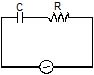Solution: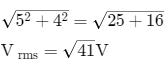QUESTION: 11

Let f = 50 Hz, and C = 100 mF in an AC circuit containing a capacitor only. If the peak value of the current in the circuit is 1.57 A at t = 0. The expression for the instantaneous voltage across the capacitor will be

Solution:

Peak value of voltage
V0=i0XC =i0/2πvC
=>1.57/(2x3.14x50x100x10-6)
Hence if equation of current i = io sin ω t then in capacitive circuit voltage is
V=V0 (ωt -  π/2)
=>50[sin2π x50t- (π/2)]
=50[100πt-(π/2)]

QUESTION: 12

In a series CR circuit shown in figure, the applied voltage is 10 V and the voltage across capacitor is found to be 8V. Then the voltage across R, and the phase difference between current and the applied voltage will respectively be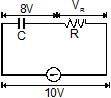Solution:

VR​=√ [V2−VC2​​]=√ [(10)2−(8)2]​=6V
tanϕ= XC/XR​ ​​= VC​​/VR​ =8/6​=4/3​

QUESTION: 13

The phase difference between current and voltage in an AC circuit is p/4 radian. If the frequency of AC is 50 Hz, then the phase difference is equivalent to the time difference :

Solution:
The period of a whole cycle at 50Hz is 1/50 = 0.02 Seconds.(This is the time it would take a generator rotor to complete one rotation).That is a full circle 2π radians (or 360 Degrees).
The phase difference (angle between curent and voltage) is π/4 part of this circle.That corresponds to 0.02 Seconds * (π/4) / (2 π) = 0.02 Seconds * 1/8 = 0.0025 Seconds.Or 2.5 milliseconds.So, the current vector leads (or lags) the voltage vector by 2.5 milli seconds.
QUESTION: 14

The given figure represents the phasor diagram of a series LCR circuit connected to an ac source. At the instant t' when the source voltage is given by V = V0coswt, the current in the circuit will be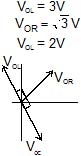Solution:
QUESTION: 15

Power factor of an L-R series circuit is 0.6 and that of a C_R series circuit is 0.5. If the element (L, C, and R) of the two circuits are joined in series the power factor of this circuit is found to be 1. The ratio of the resistance in the L-R circuit to the resistance in the C-R circuit is

Solution:

cosϕ1=0.6=5/3
tanϕ1=4/3= XC/R2…(1)
cosϕ1=0.5=1/2
tanϕ2=√3= R2/XC…(2)
From (1) and (2)3√3/4=R1/R2

QUESTION: 16

The direct current which Would give the same heating effect in an equal constant resistance as the current shown in figure, i.e. the r.m.s. current, is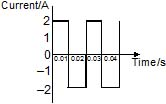Solution:
QUESTION: 17

The effective value of current i = 2 sin 100p t + 2 sin (100pt + 30º) is

Solution:

cosθ=sin(90o−θ)
sinα+sinβ=2sin (α+β/2)​cos (α−β​/2)
i=2sin100πt+2cos(100πt+30o)
=2sin100πt+2sin(90o−(100πt+30o))
=2sin100πt+2sin(60o−100πt)
=2 x 2 x sin [{(100−100)πt+60o }/2] x cox[(100+100)πt−60o/2]
​=4 x sin30o∗cos(100πt−30o)
=4x(1/2) x cos(100πt−30o)
=2cos(100πt−30o)
therefore, Io​=2A
Irms​=2 / √2​= √2​A

QUESTION: 18

A student in a lab took a coil and connected it to a 12 V DC source. He measures the steady state current in the circuit to be 4A. He then replaced the 12 V DC source by a 12 V, (w = 50 rad/s) AC source and observes that the reading in the AC ammeter is 2.4 A. He then decides to connect a 2500 mF capacitor in series with the coil and calculate the average power developed in the circuit. Further he also decides to study the variation in current in the circuit (with the capacitor and the battery in series).

Based on the readings taken by the student answer the following questions.

The value of resistance of the coil calculated by the student is

Solution:
QUESTION: 19

A student in a lab took a coil and connected it to a 12 V DC source. He measures the steady state current in the circuit to be 4A. He then replaced the 12 V DC source by a 12 V, (w = 50 rad/s) AC source and observes that the reading in the AC ammeter is 2.4 A. He then decides to connect a 2500 mF capacitor in series with the coil and calculate the average power developed in the circuit. Further he also decides to study the variation in current in the circuit (with the capacitor and the battery in series).

Based on the readings taken by the student answer the following questions.

The power developed in the circuit when the capacitor of 2500 mF is connected in series with the coil is

Solution:

VDC=IDCR
∴R=VDC/IDC=12/4=3Ω
IAC=VAC/Z=VAC√(R2+XL2)
2.4=12√ [(3)2+XL2]
solving this equation, we get,
XL=4Ω
XC=1/ωC=1/(50×2500×10−6)
=8Ω
Z=√[R2+(XC−XL)2] =5Ω
∴I=VDC/Z=12/5=2.4A=Irms
P=Irms2R=(2.4)2(3)
=17.28W
At given frequency XC>XL if omega is further decreased XC will increase (as XC∝1/ω) and XL will increase (as XL∝ω).
Therefore XC−XL and hence Z will increase. So, current will decrease.

QUESTION: 20

A student in a lab took a coil and connected it to a 12 V DC source. He measures the steady state current in the circuit to be 4A. He then replaced the 12 V DC source by a 12 V, (w = 50 rad/s) AC source and observes that the reading in the AC ammeter is 2.4 A. He then decides to connect a 2500 mF capacitor in series with the coil and calculate the average power developed in the circuit. Further he also decides to study the variation in current in the circuit (with the capacitor and the battery in series).

Based on the readings taken by the student answer the following questions.

Which of the following graph roughly matches the variations of current in the circuit (with the coil and capacitor connected in the series) when the angular frequency is decreased from 50 rad/s to 25 rad/s ?

Solution:

VDC=IDCR
∴R=VDC/IDC=12/4=3Ω
IAC=VAC/Z=VAC√(R2+XL2)
2.4=12√ [(3)2+XL2]
solving this equation, we get,
XL=4Ω
XC=1/ωC=1/(50×2500×10−6)
=8Ω
Z=√[R2+(XC−XL)2] =5Ω
∴I=VDC/Z=12/5=2.4A=Irms
P=Irms2R=(2.4)2(3)
=17.28W
At given frequency XC>XL if omega is further decreased XC will increase (as XC∝1/ω) and XL will increase (as XL∝ω).
Therefore XC−XL and hence Z will increase. So, current will decrease.

QUESTION: 21

If a direct current of value 'a' ampere is superimposed on an alternating current I = b sin wt flowing through a wire, what is the effective (rms) value of the resulting current in the circuit ?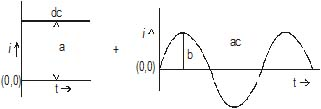Solution:
*Answer can only contain numeric values
QUESTION: 22

Find the average for the saw-tooth voltage of peak value V0 from t = 0 to t = 2T as shown in figure.Solution:
QUESTION: 23

A circuit has a coil of resistance 50 ohms and inductance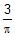henry. It is connected in series with a condenser of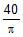m F and AC supply voltage of 200 V and 50 cycles/sec. Calculate

(i) the impedance of the circuit. (ii) the p.d. across inductance coil and condenser.

Solution:
*Answer can only contain numeric values
QUESTION: 24

A series circuit consists of a resistance, inductance and capacitance. The applied voltage and the current at any instant are given by E = 141.4 cos (5000 t _ 10°) and I = 5 cos (5000 t _ 370°) The inductance is 0.01 henry. Calculate the value of capacitance and resistance.

Solution:
QUESTION: 25

A circuit takes A current of 3 a at a power factor of 0.6 lagging when connected to a 115 V _ 50 Hz supply. Another circuit takes a current of 5A at a power factor of 0.07 leading when connected to the same supply. If the two circuits are connected in series across a 230 V, 50 Hz supply. Calculate

(a) the current (b) the power consumed and (c) the power factor.

Solution: